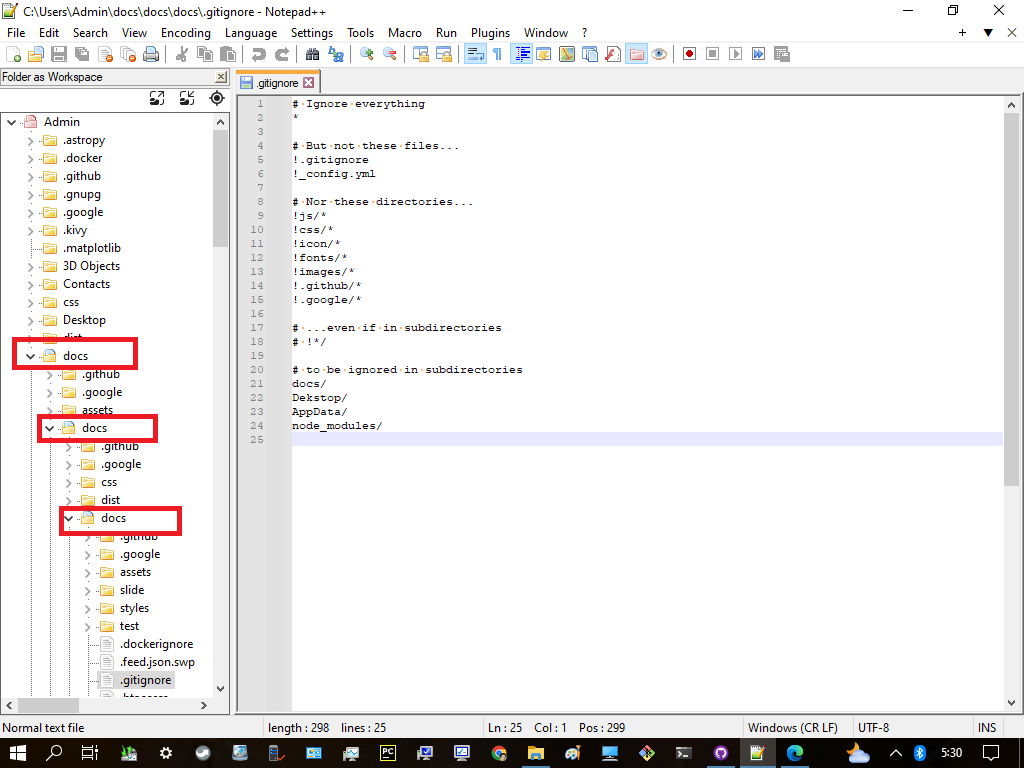Φ(10,2) = 10² + 2x(10th prime) + 10¹ = 100+29 + 29+10 = 129 + 39 = 168 = π(1000)

``````Φ = 2,10
Δ = 5,7,17
3': 13,18,25,42
2' » 13 to 77, Δ = 64
2' and 3' » 13 to 45, Δ = 32

2" + 5" = 7" = 77
2"=22, 3"=33, 2" + 3" = 5" = 55

13, 16, 18, 21, 23, 25, 28, 30, 32, 34,
36, 38, 40, 42,
45, 47, 49, 51, 53,
55, 57, 59, 61,
63, 65, 67, 69, 71, 73, 75, 77

``````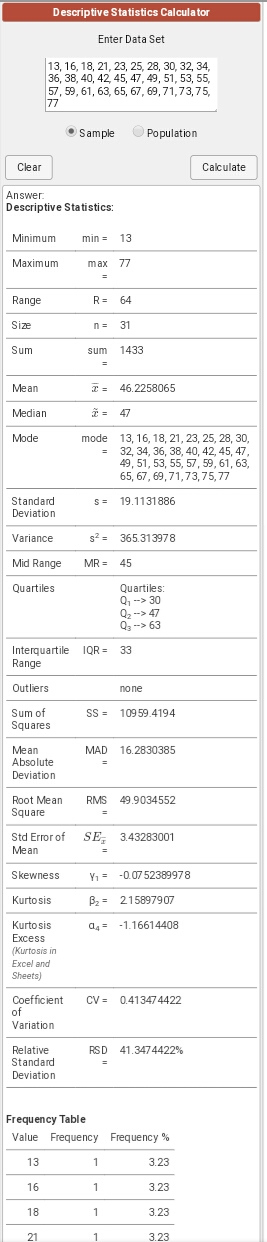The DNA base consists of 2 groups that are in pairs between Sugar and pospat with nucleotides. Let's weighting between the two. Each of these pospats consists of three (3) groups with five (5) oxygen bonds. This sugar and pospat forms a system that moves from 5' to 3' which is anti-parallel with movement at the other end which is 3' to 5'.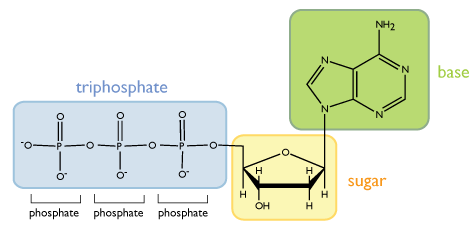Each oxygen test has 2 electrons, so each group I give a weight of 2x5 or ten (10) so the total is 30. Sugar molecules are not groups so the weight is five (5) so that with a weight of 30 out of pospat the total is 35. Since there is a pair then the total is 70. Next we unite the format with nucleotides A,T,G,C paired 9+6 and 6+9 totaling 30 then the format of both is (70,30).

``````  #8 |----------- 5® --------|------------ 7® --------------|
|  1  |---------------- 77 = 4² + 5² + 6² -------------|
-----+-----|-----+---+---+---+---+---+---+---+----+----+----+
repo| {1} | {2} | 3 | 4 | 5 | 6 | 7 | 8 | 9 | 10 | 11 |{12}| 1,77
-----+-----|-----+---+---+---+---+---+---+---+----+----+----+
user|  7  |  -  | - | - | - | 7 | 8 | - | - |  8 |  8 |  3 |
-----+-----|-----+---+---+---+---+---+---+---+----+----+----+ 7,78
main|  -  |  9  | 7 | 9 | 6 | - | - | 8 | 5 |  - |  - |  - |
-----+-----|-----+---+---+---+---+---+---+---+----+----+----+
Δ | Δ             |                       Δ  |   Δ
Φ17|Φ29            |                     96-99|  100 - 123 ({24})
|--- A,T,G,C ---|                          |  └── 100 - 103 (4x) » 100
Δ    2x2 = 4x   |-------  2x3 = 6x  -------|  └── 104 - 109 (6x) » 30
{98}                                        |  └── 110 - 123 (14x)» 70
``````

Here we unite with the whole bond which of course is the number of the two, namely 100, then the sum of the DNA bases, namely Pospat, Sugar and Nukeotides is 200 with the format (70,30,100).

(2x5x7,2x3x5,2²x5²) = (14x5,15x2,20x5) = (70,30,100)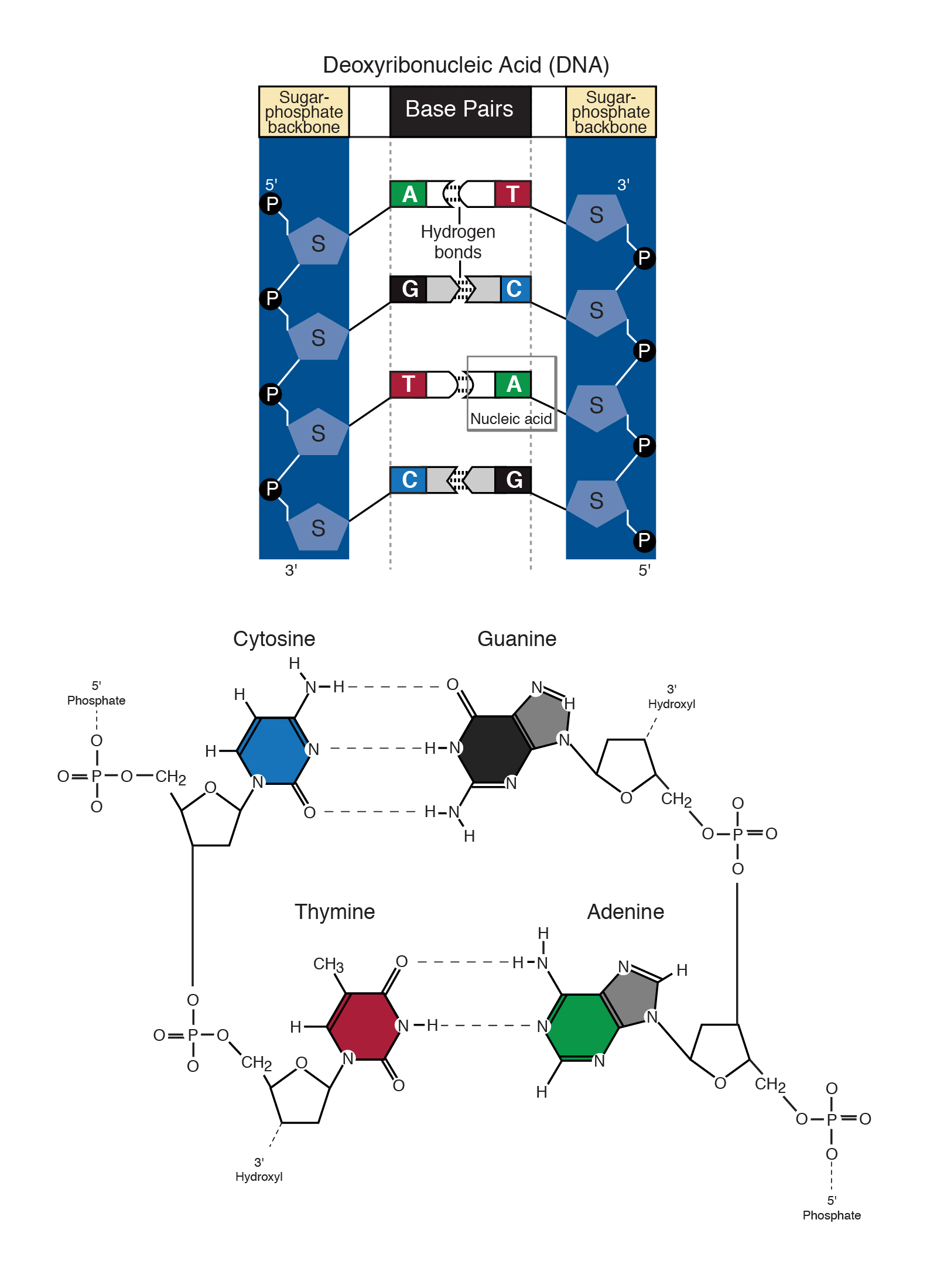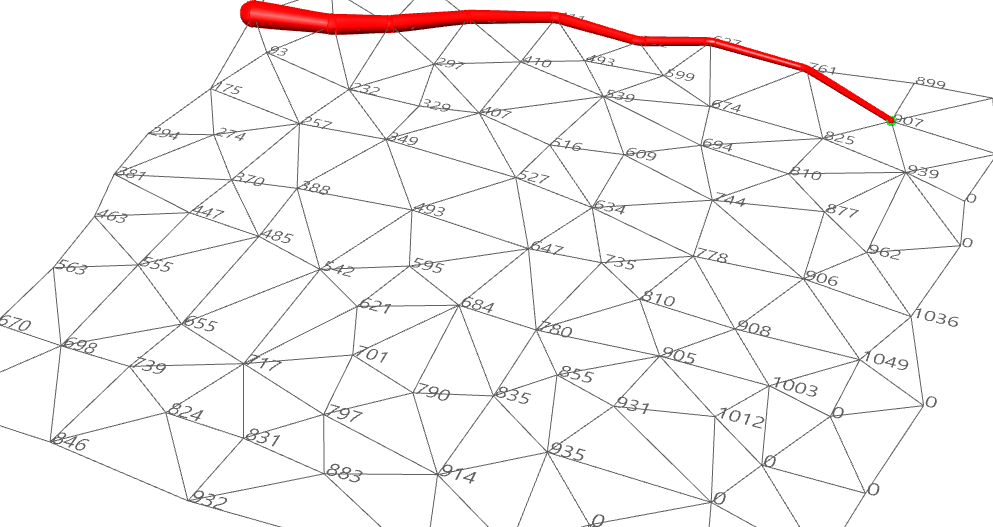114 - 102 = 12 » {1} & {2}